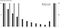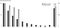Calculating CLV by summing the future cash flows to average tenure is a mistake

Why is that? After all, when calculating CLV for the average customer, it seems only natural to sum the cash flows to the average tenure, quite literally the tenure for the average customer. This is correct, but only if the cash flows in question are both the true cash flows for the average customer and undiscounted.

The “double-averaging” mistake

In an “undiscounted” world, summing the true cash flows for the average customer (that is, not reduced by the effect of churn) to the average tenure and summing the cash flows reduced over time by churn to infinity is equivalent.

Using a simple example with a constant churn rate of 25% per period, Figure 1 below shows this equivalence between doing the calculation for the average customer (“Average customer”) and summing to infinity while accounting for churn over time (“Retention to infinity”). The average tenure is 4 periods given the constant churn rate of 25% per period.Figure 1: Undiscounted “retention to infinity” vs. discounted “average customer” cash flows

However, this means that both accounting for churn and summing to the average tenure “double-averages” the calculation and is a mistake (and unfortunately a common one).

Discounting breaks the math

Which of the two methodologies should we prefer then: accounting for churn and summing to infinity, or summing the cash flows of the average customer to average tenure?

On Figure 1 above, it is clear that the cash flows of the average customer are front-loaded compared to the cash flows when accounting for churn. This means that when discounting, the value of these cash flows will be higher than the value of the cash flows when accounting for churn. The two methods are not equivalent in a discounted world: summing the cash flows to average tenure is a mistake.

Figure 2 below compares the two methods using the same example as above, and shows that using the “average customer” method overestimates CLV by 11% when the cash flows are discounted at 10% per period.Figure 2: Discounted “retention to infinity” vs. discounted “average customer” cash flows

Conclusion

The correct way to forecast CLV is to directly include the effect of churn in the calculation, and sum the cash flows to infinity.

Analytics-focused consultant at Bain & Company with a strong interest in Customer Lifetime Value. Angel investor & start-up advisor.